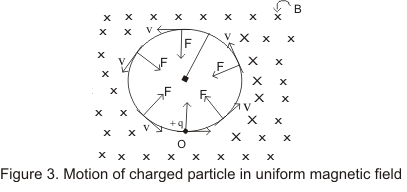# Motion of a charged particle in magnetic field

## Motion of Charged Particle in The Magnetic Field

• As we have mentioned earlier magnetic force F=(vXB) does not do any work on the particle as it is perpendicular to the velocity.
• Hence magnetic force does not cause any change in kinetic energy or speed of the particle.
• Let us consider there is a uniform magnetic field B perpendicular to the plane of paper and directed in downward direction and is indicated by the symbol C in figure shown below.• Now a charge particle +q is projected with a velocity v to the magnetic field at point O with velocity v directed perpendicular to the magnetic field.
• Magnetic force acting on the particle is
F=q(v X B) = qvBsinθ
Since v is perpendicular to B i.e., angle between v and B is θ=90 Thus charged particle at point O is acted upon by the force of magnitude
F=qvB
and the direction of force would be perpendicular to both v and B
• Since the force f is perpendicular to the velocity, it would not change the magnitude of the velocity and the effect of this force is only to change the direction of the velocity.
• Thus under the action of the magnetic force of the particle will more along the circle perpendicular to the field.
• Therefore the charged particle describe an anticlockwise circular path with constant speed v and here magnetic force work as centripetal force. Thus
F=qvB=mv2/r
where radius of the circular path traversed by the particle in the magnetic in field B is given as
r=mv/qB                   ---(5)
thus radius of the path is proportional to the momentum mv of the charged particle.
• 2πr is the distance travelled by the particle in one revolution and the period T of the complete revolution is
T=2 πr /v
From equation(5)
r/v=m/qB
time period T is
T=2πm/qB                   (6)
and the frequency of the particle is f=1/T=qB/2πm                   (7)
• From equation (6) and (7) we see that both time period and frequency does not dependent on the velocity of the moving charged particle.
• Increasing the speed of the charged particle would result in the increase in the radius of the circle. So that time taken to complete one revolution would remains same.
• If the moving charged particle exerts the magnetic field in such a that velocity v of particle makes an angle θ with the magnetic field then we can resolve the velocity in two components

vparallel : Components of the velocity parallel to field
vperpendicular :component of velocity perpendicular to magnetic field B
• The component vpar would remain unchanged as magnetic force is perpendicular to it.
• In the plane perpendicular to the field the particle travels in a helical path. Radius of the circular path of the helix is

r=mvperpendicular/qB=mvsinθ/qB                   (8)